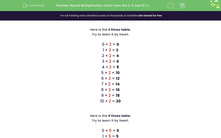# Recall Multiplication Facts from the 2, 5 and 10 Times Tables

In this worksheet, students will recall multiplication facts from the 2, 5 and 10 times tables.Key stage:  KS 1

Curriculum topic:   Number: Multiplication and Division

Curriculum subtopic:   Use Multiplication/Division Facts (2, 5, 10)

Popular topics:   Times Table worksheets

Difficulty level:#### Worksheet Overview

Here is the 2 times table.

Try to learn it by heart.

0 × 2 = 0

1 × 2 = 2

2 × 2 = 4

3 × 2 = 6

4 × 2 = 8

5 × 2 = 10

6 × 2 = 12

7 × 2 = 14

8 × 2 = 16

9 × 2 = 18

10 × 2 = 20

Once you know them by heart, you will be able to answer questions much quicker than having to work it through on your fingers!

For example, if you had to clean a whole load of football boots belonging to your friends, it might be helpful to know how many you have to clean. If 5 friends ask you to clean their boots, how many boots will you have to clean? Remember that each friend will have 2 boots!

If you don't know the 2 times table, then you could work it out by counting 5 lots of 2: 2 + 2 + 2 + 2 + 2 = 10

But it's much quicker if you do know it!      5 x 2 = 10

That's a lot of boots! Hopefully, they will all be as clean as the ones below!Here is the 5 times table.

Try to learn it by heart.

0 × 5 = 0

1 × 5 = 5

2 × 5 = 10

3 × 5 = 15

4 × 5 = 20

5 × 5 = 25

6 × 5 = 30

7 × 5 = 35

8 × 5 = 40

9 × 5 = 45

10 × 5 = 50

How about using the 5 times table to solve a problem? A shop sells tomatoes in piles of 5. How many tomatoes will you have if you buy 7 piles?

If you don't know the 5 times table, you can work out 7 lots of 5: 5 + 5 + 5 + 5 + 5 + 5 + 5 = 35

But again, it's quicker if you know it!  7 x 5 = 35Here is the 10 times table.

Try to learn it by heart.

0 × 10 = 0

1 × 10 = 10

2 × 10 = 20

3 × 10 = 30

4 × 10 = 40

5 × 10 = 50

6 × 10 = 60

7 × 10 = 70

8 × 10 = 80

9 × 10 = 90

10 × 10 = 100

Can you use it to solve this problem? Your big sister wants to paint her friends' finger nails with nail varnish. She has 6 friends to do this for. How many nails will she paint altogether? That's 6 lots of 10 to find: 10 + 10 + 10 + 10 + 10 + 10 = 60

6 x 10 = 60

I wonder what colour she's going to paint them?Let's have a go at some questions now.

### What is EdPlace?

We're your National Curriculum aligned online education content provider helping each child succeed in English, maths and science from year 1 to GCSE. With an EdPlace account you’ll be able to track and measure progress, helping each child achieve their best. We build confidence and attainment by personalising each child’s learning at a level that suits them.

Get started